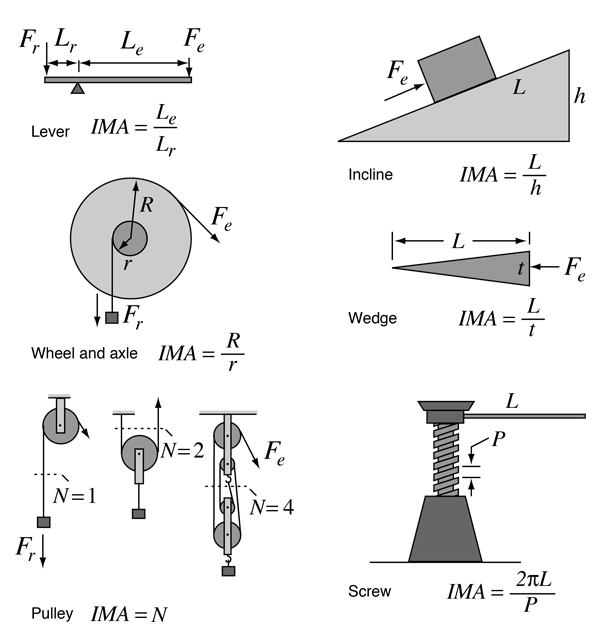# Simple Machines

It is traditional to point to a set of simple machines from which more complex machines can be fashioned. One way to characterize a "simple" machine is to say that it has no internal source of energy. It may nevertheless be very useful in that it multiply the input force to accomplish a task. The factor by which it multiplies the force is often called the "mechanical advantage". If you idealize the machine by neglecting friction, then you can state an "ideal mechanical advantage" or IMA for the machine. A typical grouping of simple machines is shown below.

Click on any machine for further details.Since we know by conservation of energy that no machine can output more energy than was put into it, the ideal case is represented by a machine in which the output energy is equal to the input energy. For simple geometries in which the forces are in the direction of the motion, we can characterize the ideal machine in terms of the work done as follows:

Ideal Machine: Energy input = Energy output

Work input = Fedinput = Frdoutput = Work output

From this perspective it becomes evident that a simple machine may multiply force. That is, a small input force can accomplish a task requiring a large output force. But the constraint is that the small input force must be exerted through a larger distance so that the work input is equal to the work output. You are trading a small force acting through a large distance for a large force acting through a small distance. This is the nature of all the simple machines above as they are shown.

Of course it is also possible to trade a large input force through a small distance for a small output force acting through a large distance. This is also useful if what you want to achieve is a higher velocity. Many machines operate in this way.

The expressions for the ideal mechanical advantages of these simple machines were obtained by determining what forces are required to produce equilibrium, since to move the machine in the desired direction you must first produce equilibrium and then add to the input force to cause motion. Both force equilibrium and torque equilibrium are applied.

 Equilibrium
Index

Torque Concepts

 HyperPhysics***** Mechanics R Nave
Go Back